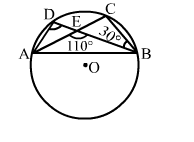# In the given figure, O is the centre of a circle and chords AC and BD intersect at E.

Question:

In the given figure, O is the centre of a circle and chords AC and BD intersect at E. If AEB = 110° and ∠CBE = 30°, then ∠ADB = ?
(a) 70°

(b) 60°
(c) 80°
(d) 90°Solution:

(c) 80°
We have:
∠AEB + ∠CEB = 180°     (Linear pair angles)
⇒ 110° + ∠CEB = 180°
∠CEB = (180° - 110°) = 70°
In
ΔCEB, we have:
∠CEB + ∠EBC + ∠ECB = 180
°   (Angle sum property of a triangle)
⇒ 70° +  30° + ∠ECB = 180°
⇒ ∠ECB = (180° - 100°) = 80°

The angles in the same segment are equal.